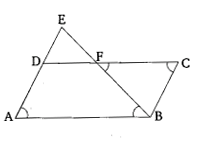# $E$ is a point on the side $AD$ produced of a parallelogram $ABCD$ and $BE$ intersects $CD$ at $F$. Show that $Ã¢Ë†â€ ABE \sim Ã¢Ë†â€ CFB$.

Given:

$E$ is a point on the side $AD$ produced of a parallelogram $ABCD$ and $BE$ intersects $CD$ at $F$.

To do:

We have to show that $∆ABE \sim ∆CFB$.

Solution:In the above figure, $ABCD$ is a parallelogram in which $E$ is a point on $AD$ produced and $BE$ intersects $CD$ at $F$.

In parallelogram $ABCD$,

$\angle A=\angle C$.......(i)           (opposite angles)

In $\triangle ABE$ and $\triangle CFB$,

$\angle EAB=\angle BCF$       (Alternate angles)

$\angle ABE=\angle BFC$

Therefore, by AA criterion,

$\triangle ABE \sim \triangle CFB$

Hence proved.